# Deep Learning papers review — NTM

Deep Learning has been an exponentially growing field in the past decade or so. And to show my enthusiasm for the field, I’m starting this series in which I’ll pick up a published paper related to deep learning (or at least something close enough) every one or two weeks, and do some sort of an analysis on the paper and give more insights into it. This way I felt I could make the community aware of some of the recent advances in Deep Learning and also arouse the curiosity of fellow Deep Learning enthusiasts.

Before I get started on today’s paper, I’d like to share this Github repo link that has a lot of Deep Learning papers listed nicely : Deep Learning Roadmap

And also, just like other programmers, I love bringing in memes all the time :P

In this article, I’ll be discussing about Neural Turing Machine (NTM), which was first released by DeepMind in the year 2014. Here’s the relevant paper : https://arxiv.org/pdf/1410.5401.pdf

In fact, NTM has been further improved by DeepMind after their initial publication and is now called the Differentiable Neural Computer (DNC), which is much more sophisticated and has really cool applications. Here’s its paper : https://www.nature.com/articles/nature20101.epdf

In this article, I’ll mostly be basing my discussions on NTM (the first one). I’ll also mention other similar work done in this field

### Neural Turing Machine

#### What is a Turing Machine ?

The Turing Machine is the most fundamental concept in computer science and the functioning of the modern day computers is derived from this. A Turing machine is an infinite tape containing slots, with a bit (either a 0 or a 1) at each slot. The bits are accessed or modified using a read or write head. The bits can be randomly accessed, erased or modified. Yet, the Turing machine is an ideal device and no “computer” is completely a Turing Machine and can perform all the tasks that a Turing Machine can perform.

#### What is a Neural Turing Machine ?

A NTM is basically a neural computer. You can think of this as the neural version of a computer as described in the Von Neumann diagram. A NTM can read and write to a memory bank just as a regular computer, but all of those operations in a NTM are differentiable. This system can be trained using backpropagation. So, just as RAM in a computer, a NTM consists of memory. And elements are read from or written to the memory using read/write heads (Inspired by Turing Machine). These read/write heads are controlled by a “Controller”. The controller is essentially just a neural network (either feed-forward or recurrent). Let’s analyze the NTM piece by piece.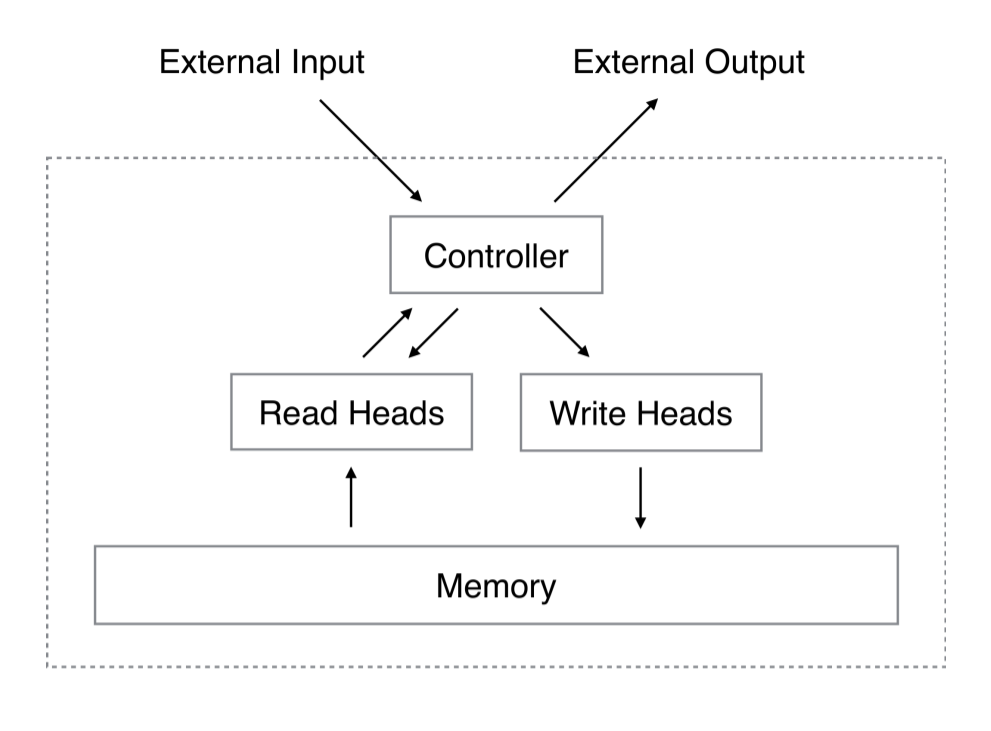Flow diagram of a NTM

#### Why NTM ?

• NTM derives a lot of inspiration from psychology, cognitive science, neuroscience and linguistics. Especially, stuff like Working memory and Attentional processes have been adopted from those fields. This is the reason for adopting the term “Neural” in the title.
• One of the reasons for introducing the NTM is the external memory. Using an external memory bank improves the pattern recognition ability of the model. You might also be wondering that a LSTM or RNN already has the ability to remember stuff from the previous time steps. Yet, implementing an external memory for this purpose is much more powerful than recurrent nets. Here’s a nice explanation for this, from this link :
Cocktail party effect: imagine that you are at a party, trying to figure out what is the name of the host while listening to all the guests at the same time. Some may know his first name, some may know his last name; it could even be to the point where guests know only parts of his first/last name. In the end, just like with a LSTM, you could retrieve this information by coupling the signals from all the different guests. But you can imagine that it would be a lot easier if a single guest knew the full name of the host to begin with.

#### Controller

The controller in a NTM is analogous to CPU in a modern day computer. This controls the read and write heads by emitting necessary vectors that lead to the formation of the weight vector. The weight vector represents the attention distribution towards the memory. It shows what parts of memory the heads focus on and by how much. As mentioned earlier, the controller is just a neural network. It could be a feed-forward net or a recurrent net. Just as any type of neural net, the controller takes in some input, gives out predictions and gets trained on the target values.

So, where do we use the data from the memory in here then ? The answer is that, the read vector from the previous time step is passed along with the data (from the dataset) as input to the controller. At every time step, the controller produces the necessary vectors to compute the weight vectors (read and write vectors have separate weights). These vectors go through a series of computations like convolution and sharpening, which we’ll see in the “Addressing mechanisms” sections below. These weight vectors are then used to read from and write to memory. Here’s a flow diagram of the controller.

#### Memory

Memory in a NTM is analogous to RAM in a modern day computer. In a NTM, memory is essentially just a matrix (a vector of vectors) from which elements are being read or to which elements are being written to. Let’s denote this matrix by M. Also let’s add a suffix ‘t’ to ‘M’, which represents the state of the matrix.

#### Read operation

Unlike a modern day computer, read operation in a NTM is differentiable. Mathematically, the read operation is just the sum of scalar multiplication of the weights and the row vectors in the memory matrix.

To get better intuition, let’s assume that each row in the memory matrix just has one element. So, the read operation now becomes equivalent to the dot product of the weight vector and elements in the memory matrix. Naturally, the elements in the memory which are being multiplied with a higher value of weight would stand out in the final product as compared to others. This means that, the attention given to various parts of the memory are different. So, some parts of the memory are more important than others. This attentional mechanism is something that the controller learns as per the input data distribution.

Here’s the read equation :

where,

• ‘r’ represents the read vector at time step ‘t’
• ‘W’ represents the weight vector at time step ‘t’
• ‘M’ represents a row vector from the memory at time step ‘t’

#### Write operation

Write operation is merely just erase + add. This is actually inspired from LSTMs as they consist of input and forget gates. The erase operation is a weighted subtraction of the erase vector from the memory. Similarly, the add operation is the weighted addition of the add vector on the memory matrix. The erase and add vectors are produced by the controller.

Let’s looking into this from a math perspective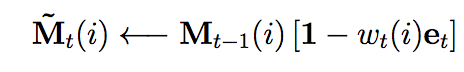Erase operation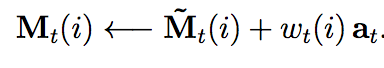Add operation

Where,

• ‘e’ is the erase vector
• ‘a’ is the add vector
• ‘w’ is the weight vector
• ‘M’ is the memory matrix

[for simplicity of implementation, you can assume the read and write weights are same ]

#### Addressing mechanism

This is the process that converts the outputs of the controller into the weight vector. There are 2 types of addressing mechanisms: Content based addressing and location based addressing. And usually in practice, we use both the types of addressing mechanisms to produce the weights.

• Content based addressing

In this type of addressing, similarity measures are produced for the elements of the memory and the Key Vector (this is one of the outputs of the controller). Let’s look into its mathematical equation :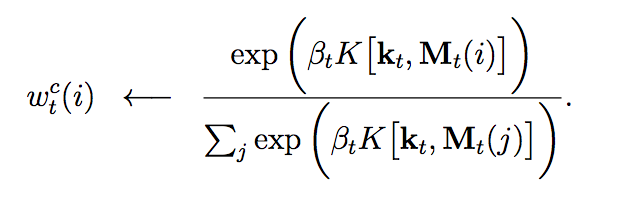This step computes the weights by content-based addressing

Where,

• ‘k’ subscripted with ‘t’ gives the Key vector at this time step — This is the vector against which we’re computing similarity measures
• K[a, b] gives the cosine similarity measure between ‘a’ and ‘b’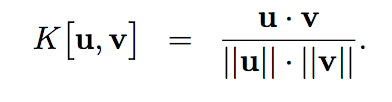Cosine similarity
• ‘β’ subscripted with ‘t’ gives the Positive key strength at time step ‘t’, which quantifies the precision of the focus. Also, ‘β’ is a controller output

The main intuition behind the content-based weights in that, they are in favor of the row in the memory matrix, which is most similar to the controller output.

• Location based addressing

This addressing mechanism involves 3 processes : interpolation, convolution and sharpening. Interpolation is the weighted sum of the content-based weights and the weights from the previous time step. In fact there is just one ‘weight’ used in that sum and it’s called the interpolation gate (g). Here’s the equation for interpolation :

Where,

• ‘g’ with a subscript of ‘t’ is the interpolation gate at time step ‘t’
• ‘w’ with superscript of ‘c’ and subscript of ‘t’ is the content-based weight at time step ‘t’
• ‘w’ with subscript ‘t-1’ is the weight from the previous time step

Convolution is the process of rotational shifting. This is done using a convolutional or a shift filter ‘s’. This shifting is analogous to head shifting in an actual Turing Machine. Let’s look at it’s math equation :

Where,

• ‘s’ subscripted with ‘t’ is the shift filter at time step ‘t’, which is given out by the controller.

The process of convolution here, is often known to cause leakage or improper dispersion of the weights. So, to mitigate this, sharpening is used. Sharpening is collectively the process of amplifying the weights by raising them to a power and normalizing them between 0 and 1. Here’s the math equation :

Where,

• ‘γ’ subscripted with ‘t’ is the sharpening factor at time step ‘t’ and ‘γ’ is always greater that or equal to 1

The use of location based addressing is that, it facilitates random access and iteration through the memory.

And in practice, we perform both the types of addressing mechanisms, as the heads should be able to use the similarity between the memory and the controller output as well as the location of the memory it focuses the most.

#### Copy Task

The copy task as the name says simply imitates the input at the final output of the controller. The final output scores of the controller here are simply compared against the input vector for optimization. Optimization is a regular neural network optimization like stochastic gradient descent. And some latent information is written to the memory while training

#### Other tasks

• Repeat copy : This requires the input to be repeated in the output a given number of times
• Associative recall : This is a task in which the network would be queried for a random element in a sequence of inputs
• Dynamic N-grams : This task is to see whether the network adopts to a given N-gram model

#### One time step of the NTM (during training)

• The controller takes in the data from the dataset as well as the read vector from the previous time step
• The controller produces the vectors required to build up weights for the read and write operation
• The controller produces the output vector (for eg., similar to the input itself in the copy task)
• Backpropagation to train the controller
• Addressing happens and weights are produced using the vectors in step 2
• Read and write operations happen and then recur to step 1

#### Final implementation

https://github.com/shravan97/Neural_Turing_Machine

[That implementation isn’t complete as yet. It’s on process]

### References

Feel free to suggest a good paper for the next post ;)